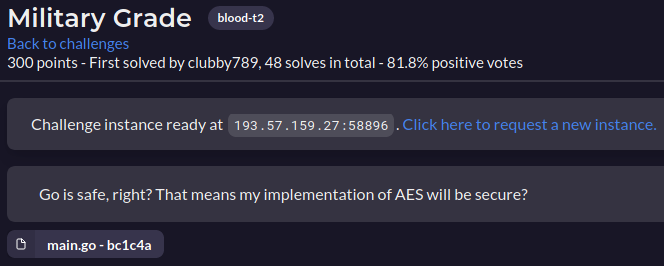# RACTF 2021 - Military Grade

Crypto-Web – 300 pts (48 solves) – Chall author: Unknown

Seeding your PRNG function with the current time is never a good idea, although using nanosecond precision might make the possible space large enough to discourage brute-forcing. It will however, get completely ruined if you use a mask such as the owner of this website. S M H The only security here is the fact that the source code is written in Go. heh take that Go!

Check out write-ups by my teammates on K3RN3L4RMY.com

## ExplorationUpon visiting the site we are greeted with a seemingly nonsensical hex string. We do note however, that the hex string changes (almost) every time we reload. Not too insightful, so time to read the source code. The following is the driver function that creates the hex string we find on the website.

func changer() {
ticker := time.NewTicker(time.Millisecond * 672).C
for range ticker {
rand.Seed(time.Now().UnixNano() & ^0x7FFFFFFFFEFFF000)
for i := 0; i < rand.Intn(32); i++ {
rand.Seed(rand.Int63())
}

var key []byte
var iv []byte

for i := 0; i < 32; i++ {
key = append(key, byte(rand.Intn(255)))
}

for i := 0; i < aes.BlockSize; i++ {
iv = append(iv, byte(rand.Intn(255)))
}

flagmu.Lock()

// The following is displayed on the website!
flag = encrypt(rawFlag, key, iv, aes.BlockSize)

flagmu.Unlock()
}
}


The ‘Ticker’ instance makes it so the code within its block is ran every ‘tick’, which in this case is set to be 672 milliseconds. That explains why the hex string changes every time we reload except for when we spam reload and catch the website twice within the same tick. Every tick, the server seeds Go’s random module and generates an IV and a key to encrypt the flag with. Let’s take a look at the server’s encrypt function.

// Apply standard PKCS5 padding
func PKCS5Padding(ciphertext []byte, blockSize int, after int) []byte {
padding := (blockSize - len(ciphertext)%blockSize)
}

// AES-CBC encryption
func encrypt(plaintext string, bKey []byte, bIV []byte, blockSize int) string {
bPlaintext := PKCS5Padding([]byte(plaintext), blockSize, len(plaintext))
block, err := aes.NewCipher(bKey)
if err != nil {
log.Println(err)
return ""
}
ciphertext := make([]byte, len(bPlaintext))
mode := cipher.NewCBCEncrypter(block, bIV)
mode.CryptBlocks(ciphertext, bPlaintext)
return hex.EncodeToString(ciphertext)
}


Quite standard, AES-CBC encryption with PKCS5 padding. So the encryption part of the server should be secure. Where is the vulnerability?

## Exploitation

If the encryption of the server is secure, there is only one other part that might be vulnerable, the PRNG. All encryption parameters are generated from Go’s random module seeded as follows.

rand.Seed(time.Now().UnixNano() & ^0x7FFFFFFFFEFFF000)


Although seeding your PRNG with the current time is never a good idea, using the time up to nanosecond precision might make brute-forcing the correct seed a bit more difficult. Possibly even beyond CTF time, as opposed to polynomial time. However, the resulting value is maked by ‘^0x7FFFFFFFFEFFF000’, where ‘^’ actually inverts the hex value to just ‘0x01000FFF’. This mask is very small and limits the possible seed space to {0 … 4095, 16777216 … 16777216+4095} for a total of 8192 values. This is definitely brute-forceable, so let’s do exactly that! Note that the Go random module does not yield the same values as Python’s random module even with the same seed, so the solve script is written in Go too.

package main

import (
"fmt"
"time"
"crypto/aes"
"crypto/cipher"
"encoding/hex"
"math/rand"
)

var encflag = "7a0f231b18f46b055d02f7e9000bd1790d6da3c19f03e1677d2cdcc9ee8c4cb9"

func decrypt(ciptext string, bKey []byte, bIV []byte, blockSize int) []byte {
bCiptext, err := hex.DecodeString(ciptext)
block, err := aes.NewCipher(bKey)
if err != nil {
fmt.Println(err)
return bKey
}
plaintext := make([]byte, len(bCiptext))
mode := cipher.NewCBCDecrypter(block, bIV)
mode.CryptBlocks(plaintext, bCiptext)
return plaintext
}

func main() {

// First range
var start int64 = 0

for k := start; k < start+4096; k++ {

rand.Seed(k)

for i := 0; i < rand.Intn(32); i++ {
rand.Seed(rand.Int63())
}

var key []byte
var iv []byte

for i := 0; i < 32; i++ {
key = append(key, byte(rand.Intn(255)))
}

for i := 0; i < aes.BlockSize; i++ {
iv = append(iv, byte(rand.Intn(255)))
}

plainhex := decrypt(encflag, key, iv, aes.BlockSize)

if (string(plainhex[:5]) == "ractf") {

fmt.Println("Gottem!")
fmt.Println(string(plainhex))

break

}

}

// Second range
start = 16777216

for k := start; k < start+4096; k++ {

rand.Seed(k)

for i := 0; i < rand.Intn(32); i++ {
rand.Seed(rand.Int63())
}

var key []byte
var iv []byte

for i := 0; i < 32; i++ {
key = append(key, byte(rand.Intn(255)))
}

for i := 0; i < aes.BlockSize; i++ {
iv = append(iv, byte(rand.Intn(255)))
}

plainhex := decrypt(encflag, key, iv, aes.BlockSize)

if (string(plainhex[:5]) == "ractf") {

fmt.Println("Gottem!")
fmt.Println(string(plainhex))

break

}

}

}


Ta-da!

ractf{int3rEst1ng_M4sk_paTt3rn}